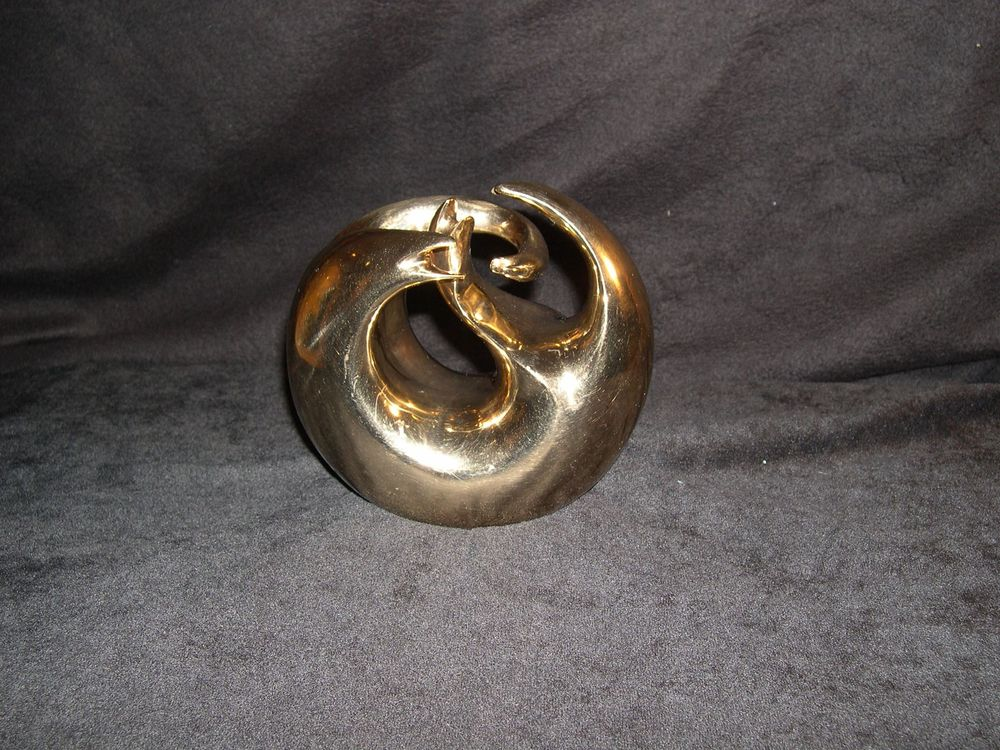# Helaman Ferguson, Sculptor

PostedDimensions: 3.5″ x 3.0″ x 1.8″ Date: 1993 Material: original stone is virginia albamarle serpentine, reproductions silicon bronze Special Engraving: the matrix (0,1 | 1,1) Weight: 4 oz Copyright Notice: © 1993 Copyright Registered: 1996.

The Fibonacci numbers are ubiquitious in nature and mathematics. This palmsize sculpture encapsulates the generating matrix for these numbers. In a problem published 800 years ago, Leonardo of Pisa, a.k.a. Fibonacci formulated his famous rabbit problem: beginning with a newborn fertile pair of rabbits, how many pairs will accumulate monthly if each pair produces another pair from their second month on? The solution of this leads to a recursively defined sequence of integers, 0, 1, 1, 2, 3, 5, 8, 13, 21, 34, 55, 89, 144, … This sequence has the property that two consequtive terms added give the next term.

The Fibonacci Matrix Torus has raised (esker) curves or continuous crests which wind around the torus either the short way or both the short and long way. This gives the matrix with first row (0, 1) and second row (1, 1) respectively. The powers of this matrix give matrices whose entries are always Fibonacci numbers.